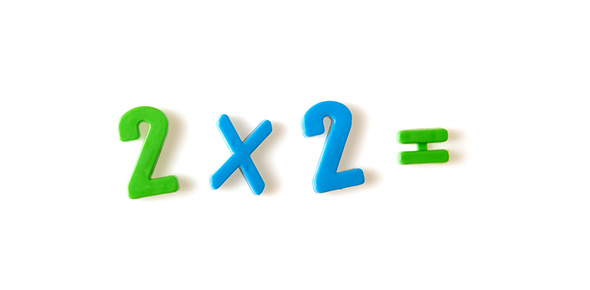# Multiplication Mixed Timed Test

90 Questions | Total Attempts: 617SettingsThis is a five minuted mixed timed multiplication facts test.

• 1.
9x1=
• 2.
5x8=
• 3.
2x5=
• 4.
7x5=
• 5.
4x7=
• 6.
0x5=
• 7.
8x6=
• 8.
0x9=
• 9.
6x3=
• 10.
7x4=
• 11.
7x0=
• 12.
4x4=
• 13.
0x3=
• 14.
6x4=
• 15.
1x7=
• 16.
3x7=
• 17.
3x1=
• 18.
5x3=
• 19.
9x9=
• 20.
9x3=
• 21.
0x4=
• 22.
7x9=
• 23.
6x0=
• 24.
3x3=
• 25.
4x8=
Related TopicsBack to top